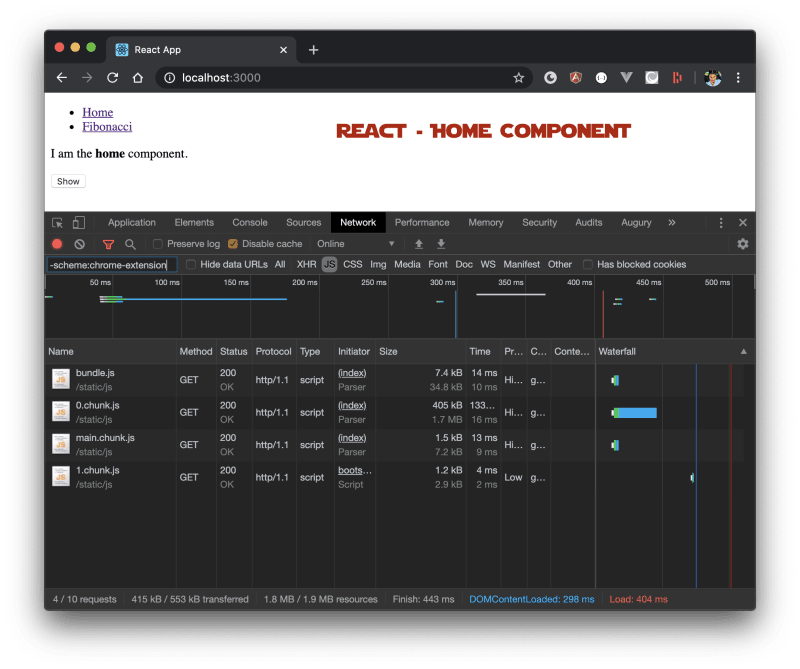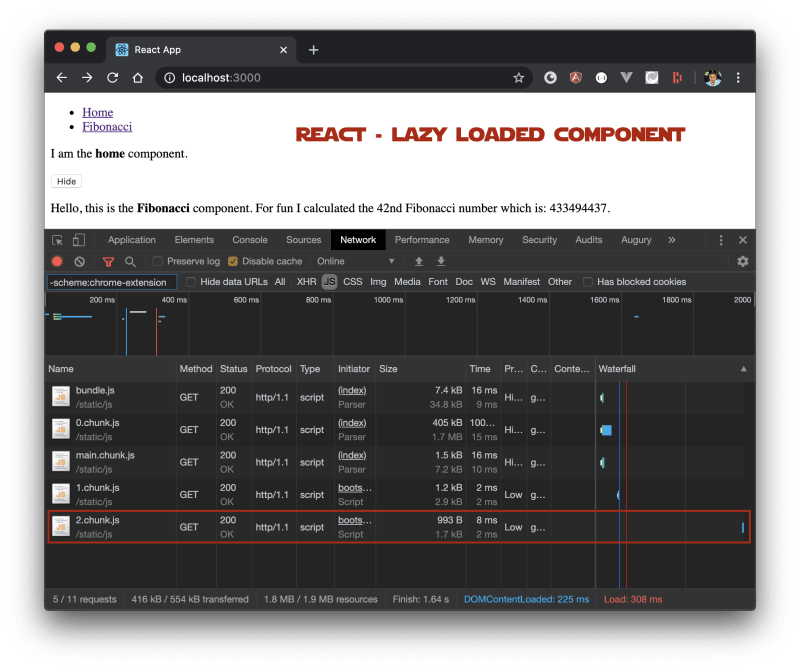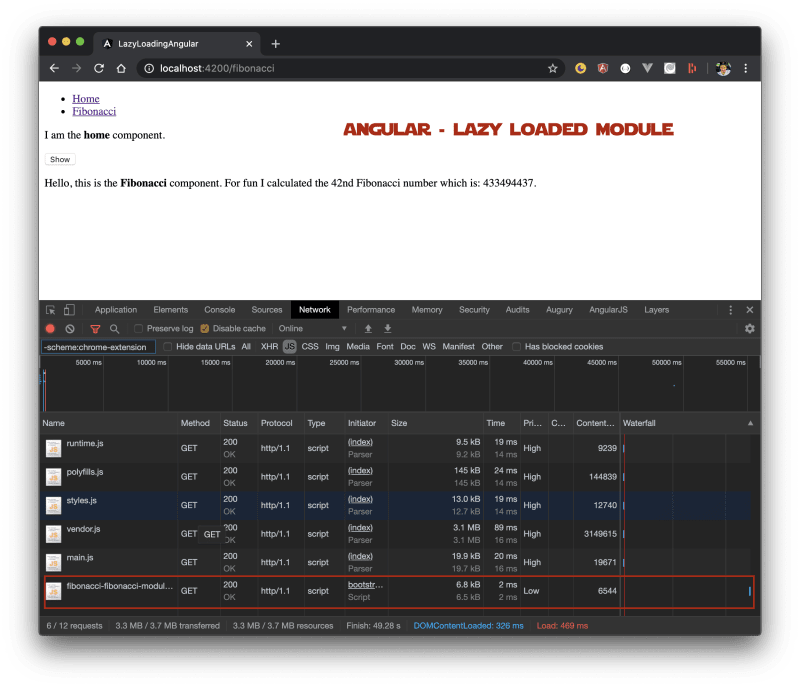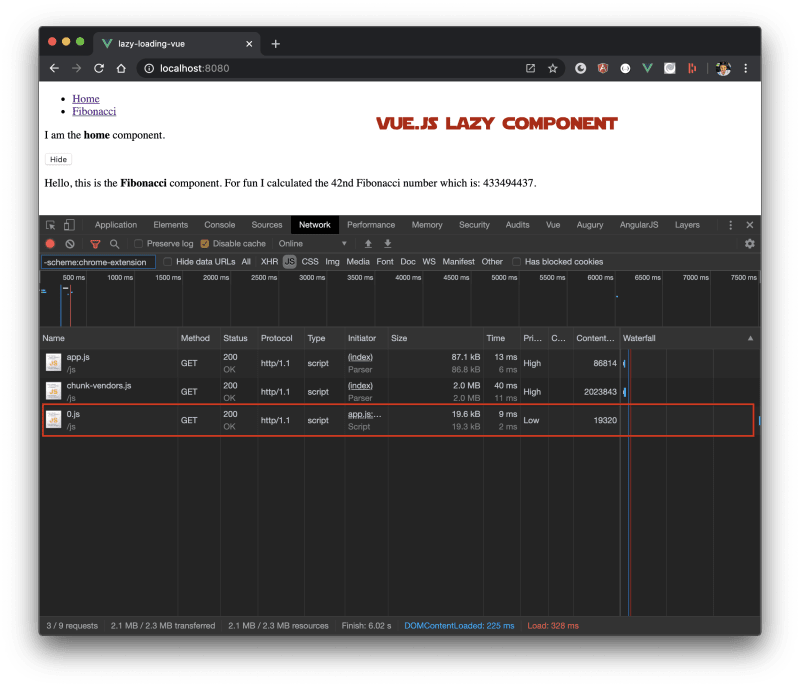Introduction

One of my favourite terms by far is "lazy-loading". Quite frankly, when a few years ago, I heard this term, it made me smile. In this article, we'll take a look at what this term means exactly for the three most used frontend frameworks today: Angular, React and Vue.js.

# Eager vs Lazy

The term eager signifies the loading of every component that we have for a give application, therefore posing potential performance bottlenecks. Lazy-loading, on the other hand, makes sure that a given component gets loaded only when it's needed and not a moment before.

The sample applications created in all three frameworks are all very similar. Each of them shows the following two things:
How to lazy-load a component via routing
To effectively visualise lazy-loading, the demo application/component is going to calculate the 42nd Fibonacci number. Mathematical operations are considered to be blocking - meaning that our programme cannot progress further; it needs to return the result of the calculation. This strategy is only being used to mimic what happens if there exists a piece of code that takes a long time to execute, and what impact it has on the overall application usage experience.

# Angular

Let's start our discussion with Angular because this frontend framework has a particularity when it comes to lazy-loading components. In fact, in Angular, the smallest logical unit that we can consider for lazy-loading via routing is a module because components always belong to modules.

## The Fibonacci component

This is how our component looks like in Angular:

``````fibonacci: number;

ngOnInit(): void {
const fibonacci = num => {
if (num <= 1) return 1;
return fibonacci(num - 1) + fibonacci(num - 2);
};
this.fibonacci = fibonacci(42);
}
``````

Since this is an individual component and it already belongs to the root Angular component, we can load it on a page.

First let's take a look at how to load the component on a page. To achieve this we have the following added to `app.component.html`:

``````<div>
<p>I am the <strong>home</strong> component.</p>
<button (click)="showMe()">{{ showFibonacci ? 'Hide' : 'Show' }}</button>
<div *ngIf="showFibonacci">
<app-fibonacci-one></app-fibonacci-one>
</div>
</div>
``````

Along with the following TypeScript code:

``````export class AppComponent {
showFibonacci:Boolean = false;
showMe() {
this.showFibonacci = !this.showFibonacci
}
}
``````

What's really interesting in this situation is the fact that the `Fibonacci` component will only get loaded if `showFibonacci` is set to `true`. This means that just using `ngIf` can be utilised for lazy-loading. This is because Angular doesn't just show/hide the component in the DOM; it adds/removes it based on the specified condition.

For discussing lazy-loading via routing in Angular, we have already established that there's a need to create a feature module.

Creating a feature module in our app along with the second component can be achieved by using the Angular CLI: `ng g m fibonacci && ng g c --module=fibonacci fibonacci`.

Once we have the module created, we can assign a component to the module, and then we need to add it to the main routing module (`app-routing.module.ts`):

``````const routes: Routes = [{
path: 'fibonacci', loadChildren: () => import('./fibonacci/fibonacci.module').then(m => m.FibonacciModule)
}];
``````

Note that we are using `loadChildren()` and import the module as part of the route definition. This means that the module will be loaded only when the appropriate route is active.

Compare the above code with the following:

``````import { FibonacciComponent } from './fibonacci/fibonacci.component';

const routes: Routes = [{
path: 'fibonacci', component: FibonacciComponent
}];
``````

The above will eagerly load the `FibonacciComponent`. It will cause a significant delay in displaying the main page of the application. Why block the main page with an operation in a component that we are not even seeing/using?

# Vue.js

Next up, let's take a look at how to achieve lazy-loading in Vue.js. Let's create a Vue.js app (using the Vue CLI) and add a new component. Take a look at how the component's `<script>` part would look like:

``````const fibonacci = num => {
if (num <= 1) return 1;
return fibonacci(num - 1) + fibonacci(num - 2);
};
const myFibonacci = fibonacci(42);

export default {
name: 'Fibonacci',
data: () => ({
fibonacci: 0
}),
created() {
this.fibonacci =myFibonacci;
}
};
``````

Note the reason why we need to do the calculation outside the `export default {}` block is that otherwise, we couldn't mimic a blocking operation. Naturally, Vue.js has both the `mounted` and `method` properties available for components, which would allow the code to be invoked only when the component is created.

With Vue.js we can utilise `v-if` to add/remove an element from the DOM and therefore lazily load a component. However there's more work that we need to do when it comes to Vue.js vs Angular. Take a look at the following code:

``````<div v-if="showFibonacci">
<Fibonacci />
</div>
<script>
import Fibonacci from './Fibonacci.vue';
export default {
name: 'Home',
data: () => ({
showFibonacci: false
}),
methods: {
showMe: function() {
this.showFibonacci = !this.showFibonacci;
}
},
components: {
Fibonacci
}
};
</script>
``````

This may seem like a logical way to do lazy-loading; however, upon opening the page, it becomes evident that the initial load time is really long. This is because the component is eagerly loaded regardless of the `v-if` condition. In other words, we are telling Vue to load all the components regardless of them being added to the DOM.

The load performance significantly changes if we make the following changes in the `<script>` element:

``````// import Fibonacci from './Fibonacci.vue';
export default {
name: 'Home',
data: () => ({
showFibonacci: false
}),
methods: {
showMe: function() {
this.showFibonacci = !this.showFibonacci;
}
},
components: {
// Fibonacci
Fibonacci: () => import('./Fibonacci.vue')
}
};
``````

By adding the import statement, inline, as part of the component's property, we are enabling lazy-loading for the `fibonacci` component. Now refreshing the app will mean that the main page loads really fast.. When the Fibonacci component is displayed on the main page, only then, we have some delay.

Lazy-loading components in Vue.js follows a similar pattern of that we have discussed earlier. Check out the router:

``````// excerpt
import Home from '../views/Home.vue';
import Fibonacci from '../views/Fibonacci.vue';

Vue.use(VueRouter);

const routes = [
{
path: '/',
name: 'Home',
component: Home
},
{
path: '/fibonacci',
name: 'Fibonacci',
component: Fibonacci
}
];
``````

The above router is something that you may have used/seen before in a Vue.js app. Even though it is functional, with a blocking operation at hand operation, you can observe the issue. If we have a blocking operation in the `Fibonacci` component, why should that block the loading time of the `Home` component, which is exactly what's happening.

To fix this particular problem, we can resort to the previously seen pattern and import the component at the route definition:

``````import Home from '../views/Home.vue';
// import Fibonacci from '../views/Fibonacci.vue';

Vue.use(VueRouter);

const routes = [
{
path: '/',
name: 'Home',
component: Home
},
{
path: '/fibonacci',
name: 'Fibonacci',
component: () => import('../views/Fibonacci.vue')
}
];
``````

Now loading the main page will not be blocked and the `fibonacci` component is only loaded when the user selects that route.

# React

Last but not least, let's take a look at how to achieve lazy-loading using React. The application was created using the `create-react-app` CLI and similarly to the previous examples, we have a component with some blocking operation:

``````import React from 'react';

const fibonacci = num => {
if (num <= 1) return 1;
return fibonacci(num - 1) + fibonacci(num - 2);
};

const fib = fibonacci(42);

const Fibonacci = () => (
<>
<p>Hello, this is the <strong>Fibonacci</strong> component. For fun I calculated the 42nd Fibonacci number which is: { fib }.</p>
</>
);

export default Fibonacci;
``````

By default, just like in the previous examples using different frameworks, importing components will mean eager loading:

``````import Fibonacci from './Fibonacci';
const Home = () => {
const [showFibonacci, setShowFibonacci] = useState(false)
const showMe = () => setShowFibonacci(!showFibonacci);
return (
<>
<p>I am the <strong>home</strong> component.</p>
<button onClick={showMe}>{ showFibonacci ? 'Hide' : 'Show' }</button>

{ showFibonacci ? <Fibonacci /> : '' }
</>
)};
``````

In the above example, even though the `Fibonacci` component is not being shown, loading the main page of the application still takes a lot of time. To fix this, we need to tell React to lazy-load the component in question. React provides a few helpers such as the `Suspense` component (for displaying a placeholder while the component is being loaded) and the `lazy()` method which load component lazily:

``````import React, { Suspense, useState, lazy } from 'react';
const Fibonacci = lazy(() => import('./Fibonacci'));
// ...
``````

Making these changes in the application will mean that the home component will load fast, and the `Fibonacci` component will only load when the user is asking for it.

The same theory applies to lazy-loading a component via routing as well - including the usage of `Suspense` and `lazy()`:

``````import Fibonacci from './components/Fibonacci';
<Router>
<nav>
<ul>
<li>
</li>
<li>
</li>
</ul>
</nav>
<Switch>
<Route exact path="/" component={Home}/>
<Route path="/fibonacci" component={Fibonacci}/>
</Switch>
</Suspense>

</Router>
``````

Given the router above, in combination with the `import` statement means that the `Fibonacci` component will be loaded eagerly. Hopefully, by now, it's clear why this is not ideal. To enable lazy-loading for components via routing, we need to change the code to utilise the aforementioned `Suspense` component and `lazy()` method:

``````// import Fibonacci from './components/Fibonacci';
const Fibonacci = lazy(() => import('./components/Fibonacci'));

// ...
<Switch>
<Route exact path="/" component={Home}/>
<Route path="/fibonacci" component={Fibonacci}/>
</Switch>
</Suspense>
``````

# Verify via DevTools

To see what's going on behind the scenes, we can utilise the browser's DevTools panel.

What is being discussed in this section is valid for all the frameworks displayed throughout the article.

Updating the code to use lazy-loading will mean that less JavaScript is downloaded, to begin with. When the component gets loaded, a new JavaScript request appears - this is the chunk that we just requested.

Take a look at the screenshots below to see a before/after state, but I also recommend that you run these samples on your own and play around with DevTools.# One more thing

Of course lazy-loading a component doesn't solve one problem: the time of execution for the "problematic" module. In our case, the component is exaggerated of course since it's doing some heavy mathematical calculation but regardless of this, users can still visit the route and face performance issues. There are certain strategies to help overcome this issue. With all the frameworks, we can use magic comments via Webpack to dynamically add `prefetch` (or `preload`) via `<link rel="prefetch" />` to a page. Just place the magic comments before the component's name, inside the `import`:

``````  const Fibonacci = lazy(() => import(
/* webpackMode: "lazy" */
/* webpackPrefetch: true */
/* webpackChunkName: "fibonacci" */ './components/Fibonacci'));
``````

The above will add a `<link rel="prefetch" as="script" href="/static/js/fibonacci.chunk.js">` element to the DOM.

# Conclusion

Lazy-loading is a concept that allows us to select which component(s) to load later in an application to allow a better performance. This is a strategy that can be chosen over eager loading, where all components are loaded at the same time causing potential performance issues. The article highlighted how to load individual components as well as how to apply lazy-loading for components via routing in three frameworks: Angular, React and Vue.js.

Author

### Tamas Piros

Developer Evangelist, experienced Technical Trainer and Google Developer Expert in Web Technologies. Active conference speaker, passionate about the latest & greatest advancements in web technologies.